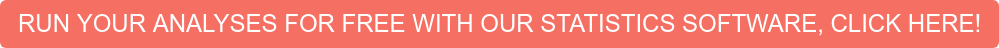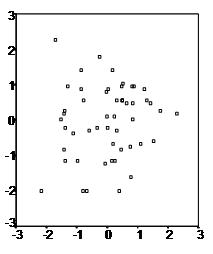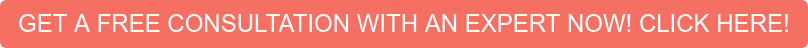# Assumptions of Multiple Linear Regression

Multiple linear regression analysis makes several key assumptions:

• There must be a linear relationship between the outcome variable and the independent variables.  Scatterplots can show whether there is a linear or curvilinear relationship.
• Multivariate Normality–Multiple regression assumes that the residuals are normally distributed.
• No Multicollinearity—Multiple regression assumes that the independent variables are not highly correlated with each other.  This assumption is tested using Variance Inflation Factor (VIF) values.
• Homoscedasticity–This assumption states that the variance of error terms are similar across the values of the independent variables.  A plot of standardized residuals versus predicted values can show whether points are equally distributed across all values of the independent variables.Intellectus Statistics automatically includes the assumption tests and plots when conducting a regression.Multiple linear regression requires at least two independent variables, which can be nominal, ordinal, or interval/ratio level variables.  A rule of thumb for the sample size is that regression analysis requires at least 20 cases per independent variable in the analysis.

Multiple Linear Regression Assumptions

First, multiple linear regression requires the relationship between the independent and dependent variables to be linear.  The linearity assumption can best be tested with scatterplots.  The following two examples depict a curvilinear relationship (left) and a linear relationship (right).Second, the multiple linear regression analysis requires that the errors between observed and predicted values (i.e., the residuals of the regression) should be normally distributed. This assumption may be checked by looking at a histogram or a Q-Q-Plot.  Normality can also be checked with a goodness of fit test (e.g., the Kolmogorov-Smirnov test), though this test must be conducted on the residuals themselves.

Third, multiple linear regression assumes that there is no multicollinearity in the data.  Multicollinearity occurs when the independent variables are too highly correlated with each other.

Multicollinearity may be checked multiple ways:

1) Correlation matrix – When computing a matrix of Pearson’s bivariate correlations among all independent variables, the magnitude of the correlation coefficients should be less than .80.

2) Variance Inflation Factor (VIF) – The VIFs of the linear regression indicate the degree that the variances in the regression estimates are increased due to multicollinearity. VIF values higher than 10 indicate that multicollinearity is a problem.

If multicollinearity is found in the data, one possible solution is to center the data.  To center the data, subtract the mean score from each observation for each independent variable. However, the simplest solution is to identify the variables causing multicollinearity issues (i.e., through correlations or VIF values) and removing those variables from the regression.

The last assumption of multiple linear regression is homoscedasticity.  A scatterplot of residuals versus predicted values is good way to check for homoscedasticity.  There should be no clear pattern in the distribution; if there is a cone-shaped pattern (as shown below), the data is heteroscedastic.If the data are heteroscedastic, a non-linear data transformation or addition of a quadratic term might fix the problem.Statistics Solutions can assist with your quantitative analysis by assisting you to editing your methodology and results chapters.  We can work with your data analysis plan and your results chapter.

Call 877-437-8622 to request a quote or email Info@StatisticsSolutions.com

Related Pages:

What is Multiple Linear Regression

Multiple Linear Regression Video Tutorial

Conduct and Interpret a Multiple Linear Regression